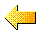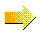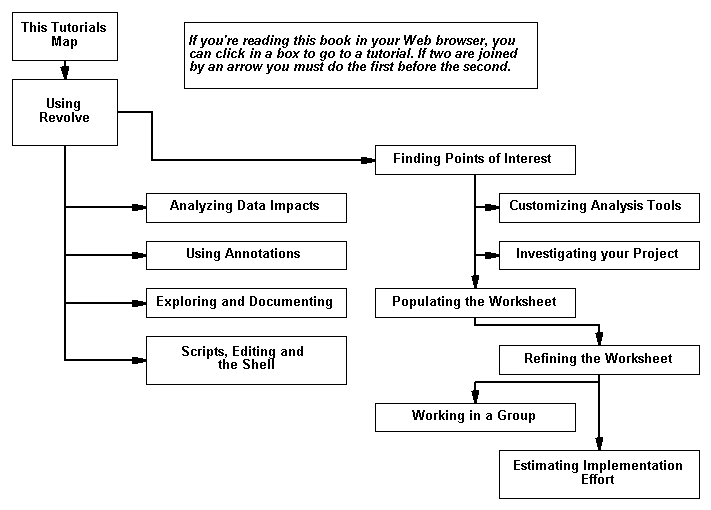Understanding the Revolve Transformation Product Suite Finding Points of Interest# Chapter 3: The Tutorials Start Here

The rest of this book is a set of tutorials in which you take existing applications and analyse them. Each tutorial is one chapter. We also use the term "session" to mean one tutorial.

## 3.1 Tutorials Map

Each tutorial is one chapter. The following diagram shows their interdependence - if two chapters are joined by an arrow you must do the first one before the second. If you are reading this book online, you can get to a chapter by clicking its box below.

These "Getting Started" tutorials are intended to give you a quick introduction to the areas they cover, by taking you through some of the most common tasks. All the features mentioned are described fully in the online help.Understanding the Revolve Transformation Product Suite Finding Points of Interest